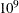# The Mixed Integer Linear Programming Solver

### Problem Statistics

Optimizers can encounter difficulty when solving poorly formulated models. Information about data magnitude provides a simple gauge to determine how well a model is formulated. For example, a model whose constraint matrix contains one very large entry (on the order of) can cause difficulty when the remaining entries are single-digit numbers. The PRINTLEVEL=2 option in the OPTMODEL procedure causes the ODS table ProblemStatistics to be generated when the MILP solver is called. This table provides basic data magnitude information that enables you to improve the formulation of your models.

The example output in Figure 7.4 demonstrates the contents of the ODS table ProblemStatistics.

Figure 7.4: ODS Table ProblemStatistics

 ProblemStatistics

Obs Label1 cValue1 nValue1
1 Number of Constraint Matrix Nonzeros 8 8.000000
2 Maximum Constraint Matrix Coefficient 3 3.000000
3 Minimum Constraint Matrix Coefficient 1 1.000000
4 Average Constraint Matrix Coefficient 1.875 1.875000
5     .
6 Number of Objective Nonzeros 3 3.000000
7 Maximum Objective Coefficient 4 4.000000
8 Minimum Objective Coefficient 2 2.000000
9 Average Objective Coefficient 3 3.000000
10     .
11 Number of RHS Nonzeros 3 3.000000
12 Maximum RHS 7 7.000000
13 Minimum RHS 4 4.000000
14 Average RHS 5.3333333333 5.333333
15     .
16 Maximum Number of Nonzeros per Column 3 3.000000
17 Minimum Number of Nonzeros per Column 2 2.000000
18 Average Number of Nonzeros per Column 2 2.000000
19     .
20 Maximum Number of Nonzeros per Row 3 3.000000
21 Minimum Number of Nonzeros per Row 2 2.000000
22 Average Number of Nonzeros per Row 2 2.000000

The variable names in the ODS table ProblemStatistics are Label1, cValue1, and nValue1.